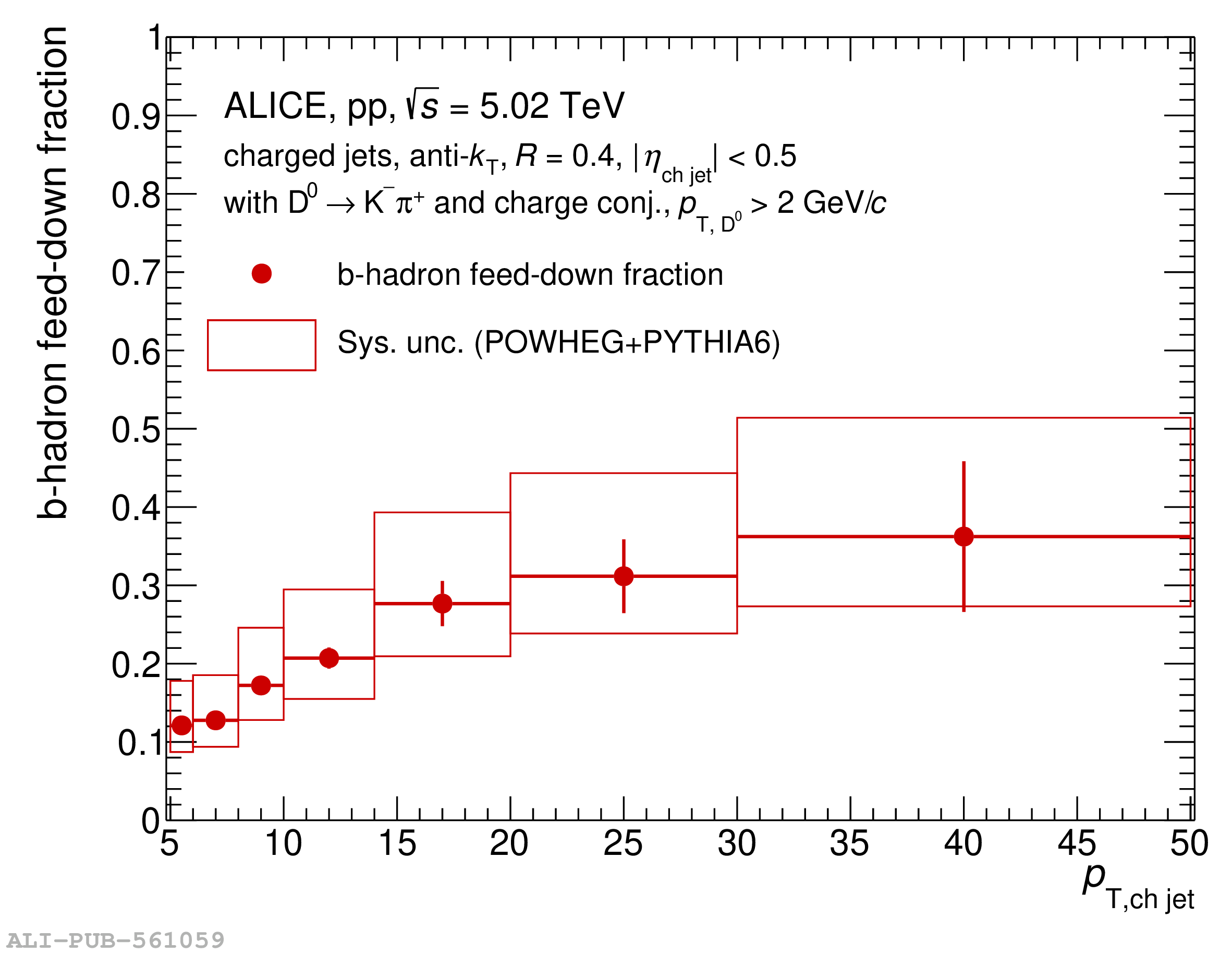# Figure 4

 Feed-down fraction of $\mathrm{D^{0}}$ jets from b-hadrons in pp collisions at $\sqrt{s}$ = 5.02 TeV for $R=0.4$ as a function of $p_{\mathrm{T,ch\,jet}}$ in $5< p_{\mathrm{T,ch\,jet}} <50$ GeV/$c$ (left) and as a function of $z_{||}^{\textrm{ch}}$ in two $p_{\mathrm{T,ch\,jet}}$ intervals $5< p_{\mathrm{T,ch\,jet}} <7$ GeV/$c$ and $15< p_{\mathrm{T,ch\,jet}} <50$ GeV/$c$ (right).